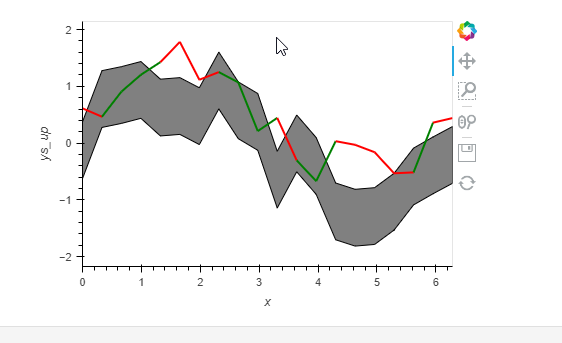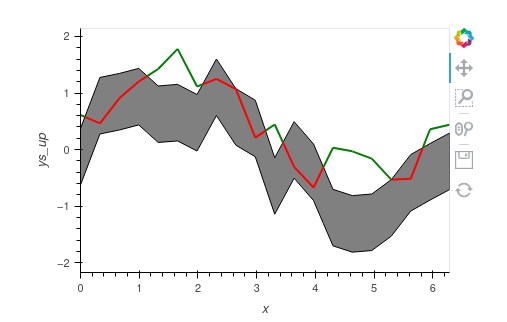# Changing line chart color when it out of a area chart

Hi

I am trying to make a chart like this:

And I try myself like this:

``````# Area example 2
import holoviews as hv
hv.extension('bokeh')
import panel as pn
pn.extension()
from holoviews import dim, opts
import numpy as np

np.random.seed(42)
xs = np.linspace(0, np.pi*2, 20) # x value
ys_up = np.sin(xs)+np.random.rand(len(xs))
ys_bo = ys_up - 1

ys_line = np.sin(xs)+np.random.rand(len(xs))
ys_color = [1 if ys_line[x]>ys_up[x] or ys_line[x]<ys_bo[x] else 0 for x in range(len(xs))]
path_data = [(xs[x],ys_line[x],ys_color[x]) for x in range(len(xs))]

levels = [0, 0.5, 1]
colors = ['green', 'red']

Area_Plot = hv.Area((xs, ys_up, ys_bo),vdims =['ys_up', 'ys_bo']).opts(color = 'gray')

Path_Plot = hv.Path([path_data], vdims='Wind Speed').opts(
color='Wind Speed', color_levels=levels, cmap=colors, line_width=2, width=450)
Area_Plot*Path_Plot

``````But this does not achieve my need, cause the color is change after a actual point not after out of the area chart.
Do we have a batter idea to make this kind of chart?

Kind regards
Victor

I don’t think there is a good way to do it other than the hard way of finding the intersection. (Maybe in geoviews). I have used shapely but another tool like scipy can also be used.

``````import holoviews as hv
import numpy as np
import panel as pn
from holoviews import dim, opts
from shapely.geometry import LineString

hv.extension("bokeh")
pn.extension()

np.random.seed(42)
xs = np.linspace(0, np.pi * 2, 20)  # x value
ys_up = np.sin(xs) + np.random.rand(len(xs))
ys_bo = ys_up - 1
ys_line = np.sin(xs) + np.random.rand(len(xs))

# Find intersections
# Ref: https://stackoverflow.com/questions/28766692/intersection-of-two-graphs-in-python-find-the-x-value
l1 = LineString(np.column_stack([xs, ys_line]))
l2 = LineString(np.column_stack([xs, ys_up]))
l3 = LineString(np.column_stack([xs, ys_bo]))

i1 = l1.intersection(l2)
i2 = l1.intersection(l3)

x1 = [p.coords.xy for p in i1.geoms] if not i1.is_empty else []
x2 = [p.coords.xy for p in i2.geoms] if not i2.is_empty else []

xsi = np.concatenate([xs, x1 + x2])
xsi.sort()

# Interpolating and finding overlap
ysi_line = np.interp(xsi, xs, ys_line)
ysi_up = np.interp(xsi, xs, ys_up)
ysi_bo = np.interp(xsi, xs, ys_bo)

olap1 = ysi_bo[:-1] + ysi_bo[1:] <= ysi_line[:-1] + ysi_line[1:]
olap2 = ysi_line[:-1] + ysi_line[1:] <= ysi_up[:-1] + ysi_up[1:]
olap = np.concatenate([olap1 & olap2, [False]])

# Creating plot
area = hv.Area((xsi, ysi_up, ysi_bo), vdims=["ys_up", "ys_bo"]).opts(color="gray")
path = hv.Path((xsi, ysi_line, olap), vdims="Wind Speed").opts(
color="Wind Speed", cmap=["green", "red"], line_width=2, width=450
)
area * path
``````3 Likes

Hi Hoxbor

This look really nice for me.

Thanks for you demo